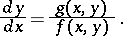# Isocline

of a first-order differential equationA set of points in the-plane at which the inclinations of the direction field defined by equation

are one and the same. Ifis an arbitrary real number, then the-isocline of equation

is the set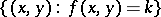(in general, this is a curve); at each of its points the (oriented) angle between the-axis and the tangent to the solution of

going through the point is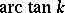. For example, the-isocline is defined by the equation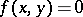and consists of just those points of the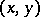-plane at which the solutions of equation

have horizontal tangents. The-isocline of

is simultaneously a solution of

if and only if it is a line with slope.

A rough qualitative representation of the behaviour of the integral curves (cf. Integral curve) of

can be obtained if the isoclines of the given equation are constructed for a sufficiently frequent choice of the parameter, and if the corresponding inclinations of the integral curves are drawn (the method of isoclines). It is also useful to construct the-isocline, defined by the equation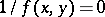; at the points of the-isocline the integral curves of equation

have vertical tangents. The (local) extreme points of the solutions of

can lie on the-isocline only, and the points of inflection of the solution can lie only on the curve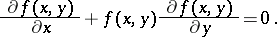For a first-order equation not solvable with respect to the derivative,the-isocline is defined as the setIn the case of a second-order autonomous system,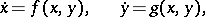the set of points in the phase plane at which the vectors of the phase velocity are collinear is an isocline of the equation